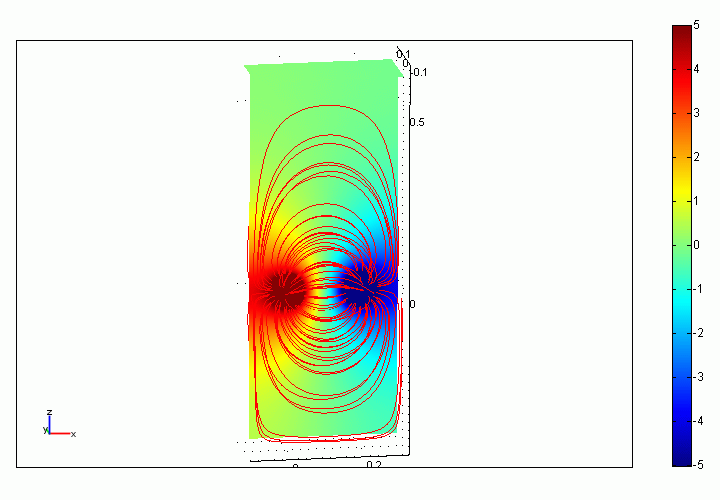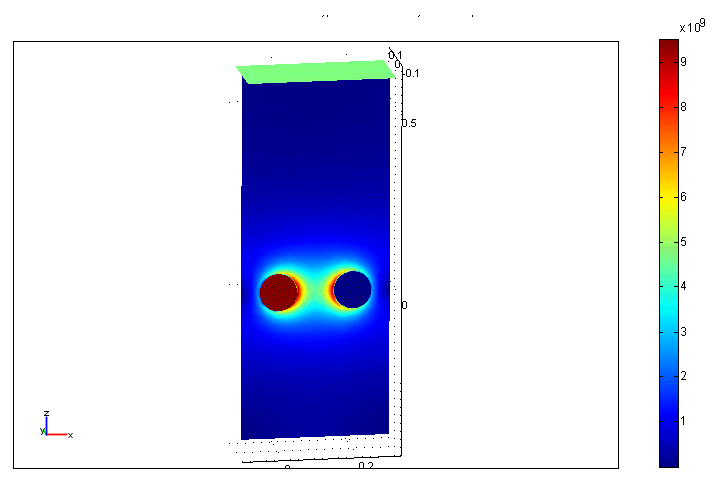# two ground balls deep in the ground close to each other$R = \frac{U}{I}$ $\varphi = \frac{I}{4 \pi \sigma} \frac{1}{r}$ $U = \varphi_2 - \varphi_1$ $R = \frac{1}{2 \pi \sigma} \Big( \frac{1}{a} - \frac{1}{s} \Big)$